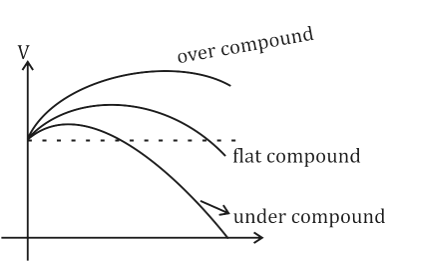Engineering Jobs   »   Electrical Engineering quizs   »   NHPC JE Recruitment 2021, NHPC JE...

# NHPC-JE’21 EE: Daily Practices Quiz 16-Sep-2021

## NHPC JE Recruitment 2021

National Hydroelectric Power Corporation (NHPC) has released the recruitment notification for engagement of following posts such as Senior Medical Officer, Assistant Rajbhasha Officer, Junior Engineer & Sr. Accountant posts. NHPC expects application from High Performing, Dynamic & Achievement-Oriented Professionals candidates to fill up these 173 vacancies.

## NHPC-JE’21 EE: Daily Practices Quiz

NHPC-JE’21 EE: Daily Practices Quiz 16-Sep-2021

Each question carries 1 mark.
Negative marking: 1/4 mark
Total Questions: 06
Time: 06 min.

Q1. A current wave is given by i = 4 + 2√2 sin 3θ + 4√2 sin 5θ. The r.m.s. value of current wave is ………
(a) 10 A
(b) 6 A
(c) √56 A
(d) 5 A

Q2. The pressure coil of an energy meter is…….
(a) Purely resistive
(b) Purely inductive
(c) Highly resistive
(d) Highly inductive

Q3. Which of the following generator has zero percentage regulation?
(a) Flat compound
(b) Over compound
(c) Under compound
(d) Both flat compound and under compound

Q4. A dc generator efficiency is maximum when:
(a) Variable loss + constant loss=1
(b) Variable loss – constant loss =1
(c) Constant loss – variable loss =1
(d) Variable loss – constant loss =0

Q5. The maximum demand of a consumer is 2 kW and the corresponding daily energy consumption is 30 units. What is the corresponding load factor?
(a) 25%
(b) 50%
(c) 62.5%
(d) 75%

Q6. A 40 KVA transformer has a core loss of 400 W and full-load copper loss of 800 W. The fraction of rated load at maximum efficiency is
(a) 50 %
(b) 62.3 %
(c) 70.7 %
(d) 100 %

## SOLUTIONS

S1. Ans.(b)
Sol. Ir.m.s. = √((4)^2+(2)^2+(4)²) = 6A

S2. Ans.(d)
Sol. The pressure coil of an energy meter is highly inductive while for wattmeter it is highly resistive.

S3. Ans.(a)
Sol. For flat compound: V_fl = V_nl i.e regulation = zeroS4. Ans.(d)
Sol. A dc generator efficiency is maximum when: Variable loss = constant loss.

S5. Ans.(c)
Sol.
30 units = 30 kWh
In 24 hours, energy consumption=30 KWh
Energy consumed in 1 hour
= 30/24 = 1.25 kW